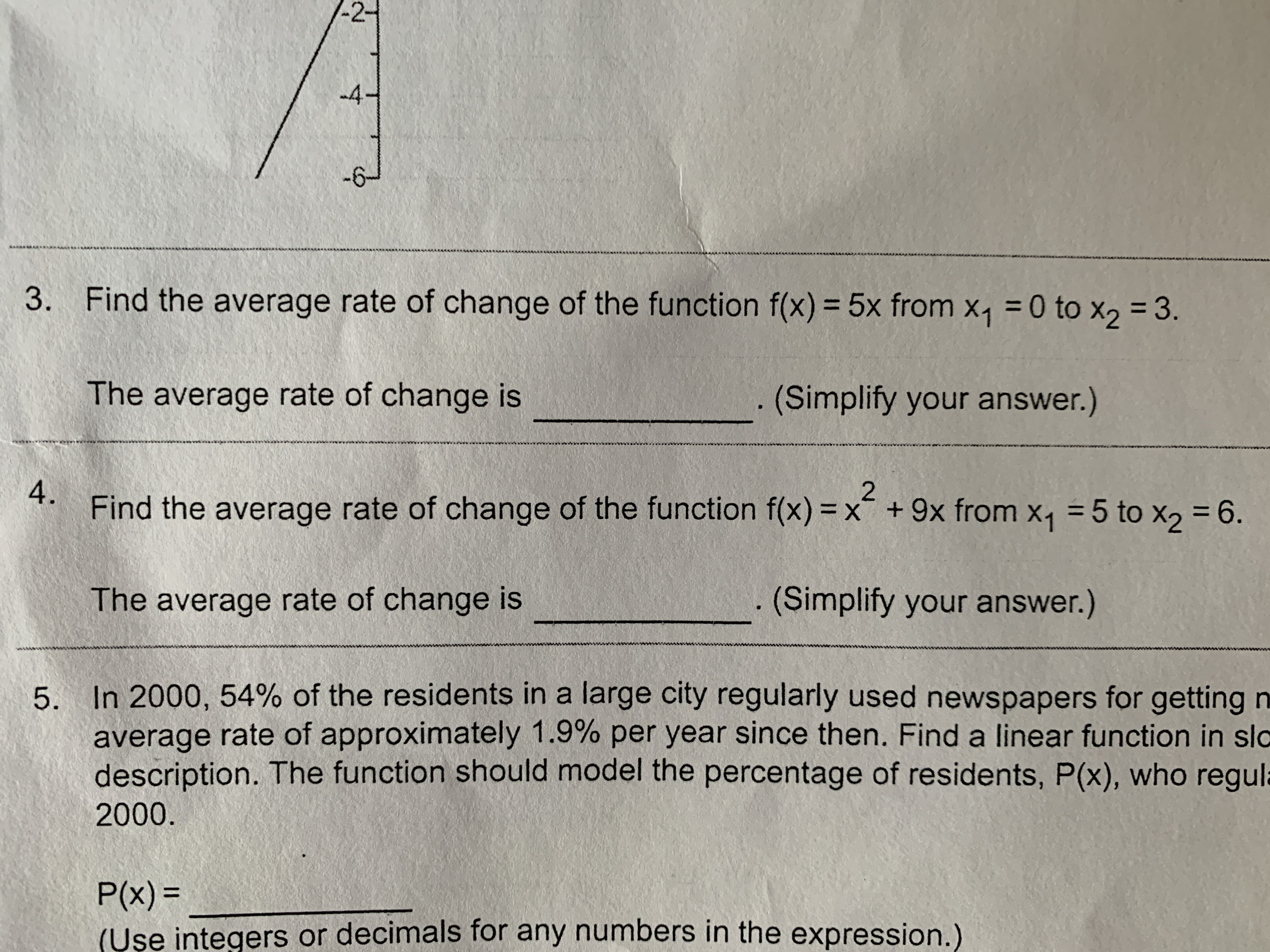# -24-63.Find the average rate of change of the function f(x) = 5x from x1 0 to x2 3.The average rate of change is(Simplify your answer.)A. Find the average rate of change of the function f(x) = x +9x from X1 = 5 to X2 = 6.The average rate of change is(Simplify your answer.)In 2000, 54% of the residents in a large city regularly used newspapers for getting naverage rate of approximately 1.9% per year since then. Find a linear function in slcdescription. The function should model the percentage of residents, P(x), who regula5.2000.P(x)=(Use integers or decimals for any numbers in the expression.)

Question
4 views

Can someone help me with this problem ?  It is problems three and fohelp_outlineImage Transcriptionclose-2 4 -6 3. Find the average rate of change of the function f(x) = 5x from x1 0 to x2 3. The average rate of change is (Simplify your answer.) A. Find the average rate of change of the function f(x) = x +9x from X1 = 5 to X2 = 6. The average rate of change is (Simplify your answer.) In 2000, 54% of the residents in a large city regularly used newspapers for getting n average rate of approximately 1.9% per year since then. Find a linear function in slc description. The function should model the percentage of residents, P(x), who regula 5. 2000. P(x)= (Use integers or decimals for any numbers in the expression.) fullscreen
check_circle

Step 1

We’ll answer the first question since the exact one wasn’t specified. Please submit a new question specifying the one you’d like answered

Step 2

Given,

...

### Want to see the full answer?

See Solution

#### Want to see this answer and more?

Solutions are written by subject experts who are available 24/7. Questions are typically answered within 1 hour.*

See Solution
*Response times may vary by subject and question.
Tagged in

### Other